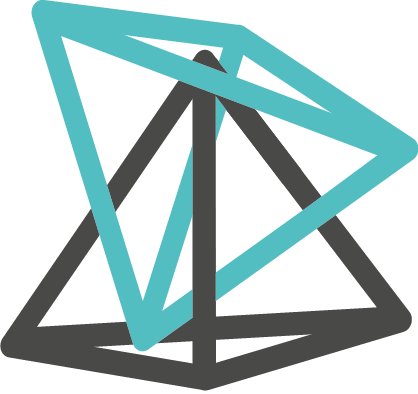# IceSL cheatsheet

### Lua

`Cheat sheet 1` `Cheat sheet 2`

### Vectors and Matrices

``v (x, y [,z]) | v {x, y [,z]}v.x|y|zm (n11, n12, ..., n44) | m {n11, n12, ..., n44}m:at(c,r)``

### Operations

let v, m and n be a vector, a matrix and a number respectively:

``v + v ; v - v ; v * v ; v / v ; v * n ; v / n ; -vm * m ; m * vdot (v, v)cross (v, v)normalize (v)length (v)inverse (m)``

### Constants and trig functions

``Pi ; X ; Y ; Zsin | cos | tan | asin | acos | atan | atan2 (degrees)``

### Emiting

``emit (shape [, brush])set_brush_color (brush, r, g, b)``

### Primitives Shapes

``load | loadmesh | load_centered | load_centered_on_plate (path)sphere(radius [, center])cube | ccube | box (d | dx, dy, dz | v )cone | ccone (r0, r1, h | r0, r1, c0, c1)cylinder | ccylinder (r, h | r, c0, c1])polyhedron ({points}, {indices})Void``

### Non-primitive Shapes

``linear_extrude (dir, {points})linear_extrude_from_oriented (dir, {points})rotate_extrude ({points}, nsteps)sections_extrude (contours)``

### Implicits

``implicit_distance_field (boxMin, boxMax, fragment)implicit_distance_field_from_file (boxMin, boxMax, fileName)implicit_solid (boxMin, boxMax, voxSize, fragment)implicit_solid_from_file (boxMin, boxMax, voxSize, fileName)set_uniform_boolean|scalar|vector|matrix|texture3d (implicit, var_name, var_value)enable_progressive_rendering (implicit, bool)GLSL cheat sheet``

### 3D textures

``tex3d_rgb32f|r32f|rgb8f|r8f (w, h, d)  :set(u, v, w, value)``

### Transformations

``translate (dx, dy, dz | v)rotate (angle, axis | rx, ry, rz | v)scale (scale | sx, sy, sz | v)mirror (direction)frame (direction)``
let T and s be a transformation and a shape respectively;
``s' = T * s``

### Magnets

``magnet (name)snap (shape0, magnet0, shape1, magnet1)``

### CSG Operations

``union | U (shape0, shape1)union | U {shape0, ..., shapeN}intersection | I (shape0, shape1)intersection | I {shape0, ..., shapeN}difference | D (shape0, shape1)difference | D {shape0, ..., shapeN}``

### Other Operations

``merge (s0, s1) | merge {s0, ..., sn}flip (mesh)convex_hull (shape)dilate (mesh, factor)erode (mesh, factor)linear_offset (mesh, direction, offset)linear_offsets (mesh, {directions}, {offsets})``

### Shape Representation

``````to_voxel_solid (shape, voxSize)to_voxel_distance_field (shape, voxSize)
set_distance_field_iso (voxels, threshold)smooth_voxels | smooth_voxels_preserve_volume (voxels, windowSize)to_mesh (shape, voxSize)voxelize_to_file (shape, voxSize, fileName)distribute (shape, density)``````

### Shapes from asset

``load_raw_voxels (fileName, thicken)svg_ex (svg_file, dpi)  :outline() :fill() :stroke() :strokeWidth() :hasFill() :hasStroke()font (ttf_file)  :str(string, tracking)``

### Tweaks and Fields

``ui_scalar (name, default, min, max)ui_field (name, boxMin, boxMax)ui_field_value_at (name, p)ui_file (fileName)``

### Mesh Information

``bbox (shape)  :min_corner() :max_corner() :center() :extent() :empty() :enlarge()mesh_vertices (mesh)mesh_normals (mesh)mesh_local_edge_size (mesh)``

### Misc

``enable_variable_cache = true | falsescreenshot ()set_tesselation_factor (factor)sleep (ms)dump (shape, filename)``